# SAT Math Multiple Choice Question 200: Answer and Explanation

### Test Information

Question: 200

5. Which of the following expressions is equivalent to (4s)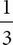?

• A.• B.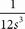• C. 2• D.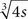DTaking a number to the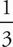power is the same as taking the cube root of the number. Therefore, (4s)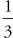=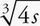. The correct answer is (D). A shortcut is to just raise the coefficient to the power, and then use POE. Since only one answer starts with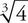, that one must be correct. Another approach is to plug in a number for s and let the calculator do the hard work, though that would not help in this section since calculator use is not permitted.